# Concept of Simple Linear Regression

Simple linear regression is used to estimate the relationship between two variables, where the variables are continuous in nature.  so it used to define relation between single input variable and single output variable and also defines how this relation can be presented by straight line.

Below plot shows the graphical relation between two continuous variable.This scatter shows three terms –

1. The direction
2. The strength
3. The linearity
Here plot shows that variable x and y shares positive liner relationship.  So the most exact way to define this data is straight line.
if relationship between two variables x and y stands strong then we can predict output variable y on the basic of input x variable nature. And this can be represented by straight line.
Now we have correlation coefficient (r) to check collinearity between variable X and Y.
correlation coefficient (r)stands for numerical value of correlation between two variables. if value of r is higher then it means that the input variable x is good for y.
During this we have to count on some properties of ‘r’, listed below-
1. Range of r: -1 to +1
2. Perfect positive relationship: +1
3. Perfect negative relationship: -1
4. No Linear relationship: 0
5. Strong correlation: r > 0.85 (depends on business scenario)

Here, Command used for calculation “r” in RStudio is:

> cor(X, Y)

where,

X: independent variable

Y: dependent variable

Now, there are two conditions depends on value of ‘r’ ,i.e result of above equation.

case 1- if r > 0.85 then choose simple linear regression and

case 2- If r < 0.85 then use transformation of data to increase the value of “r” and then build a simple linear regression model on transformed data.

There are four steps to Implement Simple Linear Regression:

1. Analyze data (analyze scatter plot for linearity)
2. Get sample data for model building
3. Then design a model that explains the data
4. And use the same developed model on the whole population to make predictions.

The equation that represents how an independent variable X is related to a dependent variable Y.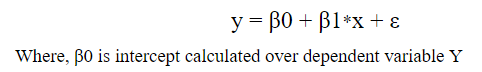For Example:

Let’s Consider we want to calculate the weight gain based upon calories taken. And for this we have below data.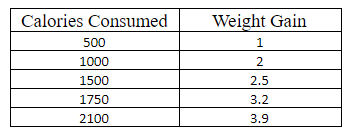here we want to know weight gain when you consume 2500 calories. First, we need to draw a  graph of the data which will show that calories consumed is  independent variable X to predict dependent variable Y.here “r”  can be calculated as follows: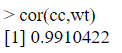As mentioned above case 1 here, r = 0.9910422 which is greater than 0.85, we can consider calories consumed as the best independent variable(X) and weight gain(Y) as the predict dependent variable.

Now, if we  try to draw a line  in a way that it should be close to every data point in the above plot diagram. It will be like this-To calculate the weight gain for 2500 calories, simply extend the straight line further to the y-axis at a value of 2,500 on x-axis . This projected value of y-axis gives you the rough weight gain. This straight line is a regression line.

Similarly, if we substitute the x value in equation of regression model such as: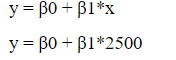y value will be predicted.

Following is the command to build a linear regression model.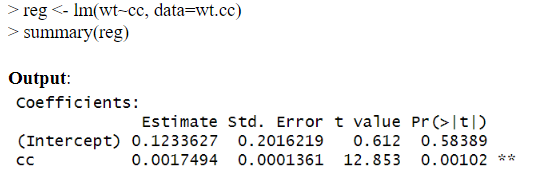We obtain the following values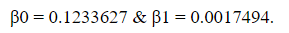Substitute these values in the equation to get y as shown below.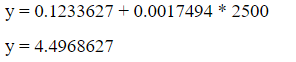So, weight gain predicted by our simple linear regression model is 4.49Kgs after consumption of 2500 calories.

Resource Box

we hope we got the detail idea about Simple Liner Regression through this blog. This blog is inspired by Excelr Solution.